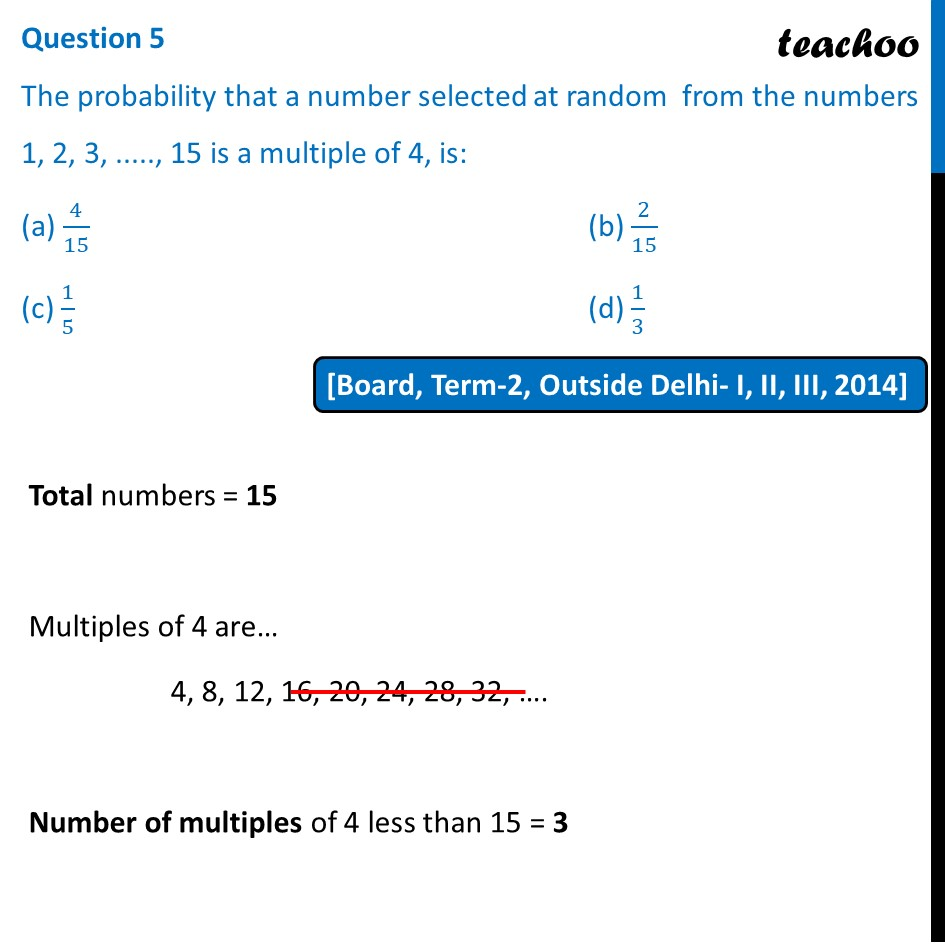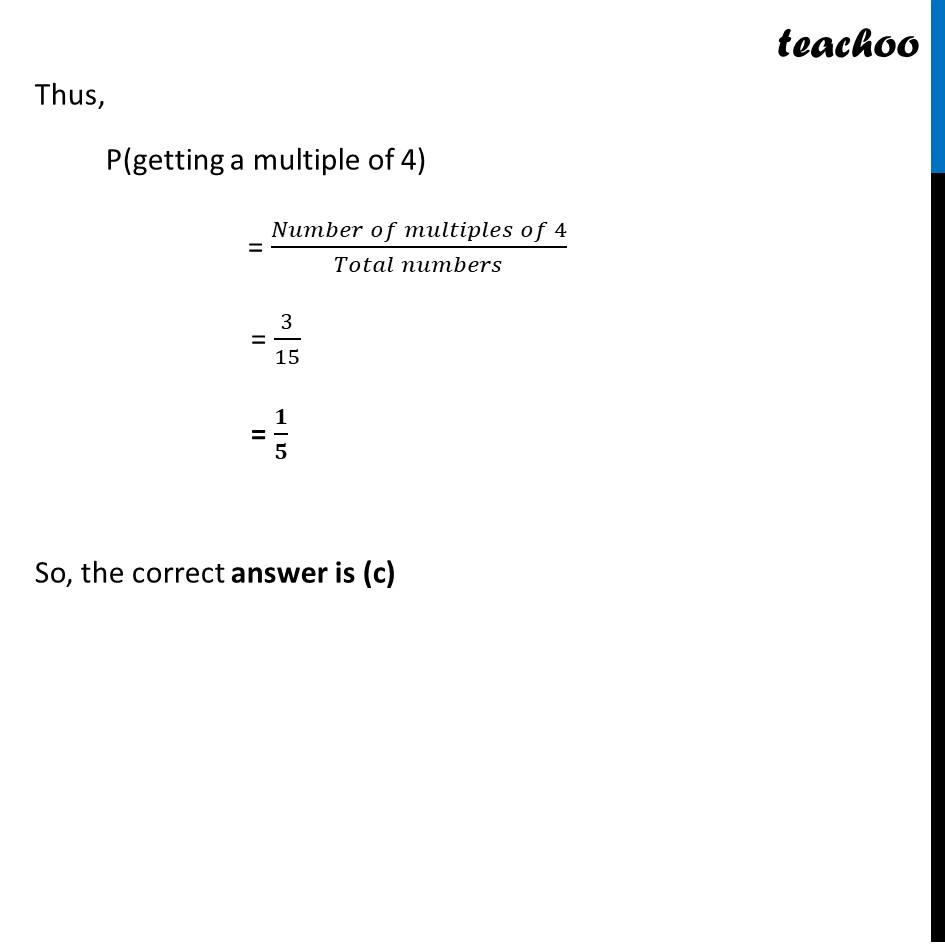Past Year MCQ

Chapter 14 Class 10 Probability
Serial order wise

## (c) 1/5                                                             (d) 1/3Learn in your speed, with individual attention - Teachoo Maths 1-on-1 Class

### Transcript

Question 5 The probability that a number selected at random from the numbers 1, 2, 3, ....., 15 is a multiple of 4, is: (a) 4/15 (b) 2/15 (c) 1/5 (d) 1/3 Total numbers = 15 Multiples of 4 are… 4, 8, 12, 16, 20, 24, 28, 32, …. Number of multiples of 4 less than 15 = 3 Thus, P(getting a multiple of 4) = (𝑁𝑢𝑚𝑏𝑒𝑟 𝑜𝑓 𝑚𝑢𝑙𝑡𝑖𝑝𝑙𝑒𝑠 𝑜𝑓 4)/(𝑇𝑜𝑡𝑎𝑙 𝑛𝑢𝑚𝑏𝑒𝑟𝑠) = 3/15 = 𝟏/𝟓 So, the correct answer is (c)